## Saturday, 9 August 2014

### CHAPTER 4-Worked Out Examples 2

 Example: 6
 Two lines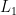${L_1}$ and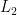${L_2}$ have direction cosines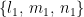$\left\{ {{l_1},\,{m_1},\,{n_1}} \right\}$ and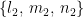$\left\{ {{l_2},\,{m_2},\,{n_2}} \right\}$respectively. Find the angle at which${L_1}$ and${L_2}$ are inclined to each other respectively.
 Solution: 6
The unit vectors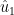${\hat u_1}$ and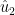${\hat u_2}$ along${L_1}$and${L_2}$ respectively can be written as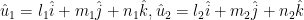${\hat u_1} = {l_1}\hat i + {m_1}\hat j + {n_1}\hat k, {\hat u_2} = {l_2}\hat i + {m_2}\hat j + {n_2}\hat k$
The angle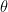${\rm{\theta }}$ between${\hat u_1}$ and${\hat u_2}$ (and hence${L_1}$ and${L_2}$ ) is given by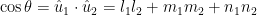$\cos \theta = {\hat u_1} \cdot {\hat u_2} = {l_1}{l_2} + {m_1}{m_2} + {n_1}{n_2}$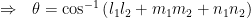$\Rightarrow \,\,\,\, \theta = {\cos ^{ - 1}}\left( {{l_1}{l_2} + {m_1}{m_2} + {n_1}{n_2}} \right)$
We can dedude the following conditions on the direction cosines of${L_1}$ and${L_2}$.
If${L_1}$ and${L_2}$ are parallel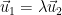${\vec u_1} = \lambda {\vec u_2}$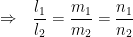$\Rightarrow\,\,\,\,{\dfrac{{{l_1}}}{{{l_2}}} = \dfrac{{{m_1}}}{{{m_2}}} = \dfrac{{{n_1}}}{{{n_2}}}}$
If${L_1}$ and${L_2}$ are perpendicular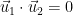${\vec u_1} \cdot {\vec u_2} = 0$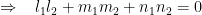$\Rightarrow\,\,\,\,\,{{l_1}{l_2} + {m_1}{m_2} + {n_1}{n_2} = 0}$
What will be the corresponding conditions had a set of direction ratios been specified instead of the direction cosines?
 Example: 7
 For the lines${L_1}$ and${L_2}$ of the previous example, find the direction cosines of the line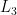${L_3}$ perpendicular to both${L_1}$ and${L_2}$ .
 Solution: 7
Let the unit vector along${L_3}$ be${\vec u_3}.$ We have,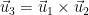${\vec u_3} = {\vec u_1} \times {\vec u_2}$$= \left| {\begin{array}{*{20}{c}} {\hat i}\,\,\,\,{\hat j}\,\,\,\,{\hat k}\\ {{l_1}}\,\,\,\,{{m_1}}\,\,\,\,{{n_1}}\\ {{l_2}}\,\,\,\,{{m_2}}\,\,\,\,{{n_2}} \end{array}} \right|$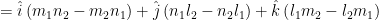$= \hat i\left( {{m_1}{n_2} - {m_2}{n_1}} \right) + \hat j\left( {{n_1}{l_2} - {n_2}{l_1}} \right) + \hat k\left( {{l_1}{m_2} - {l_2}{m_1}} \right)$
Since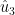${\hat u_3}$ is a unit vector itself, the direction cosines of${L_3}$ are simply$\left( {{m_1}{n_2} - {m_2}{n_1}} \right),\left( {{n_1}{l_2} - {n_2}{l_1}} \right),\left( {{l_1}{m_2} - {l_2}{m_1}} \right)$
 Example: 8
Find the angle between the lines whose direction cosines are given by the equations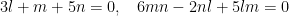$3l + m + 5n = 0,\,\,\,\,\,6mn - 2nl + 5lm = 0$
 Solution: 8
Using the value of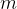$m$ from the first equation in the second, we have$- 6\left( {3l + 5n} \right)n - 2nl - 5l\left( {3l + 5n} \right) = 0$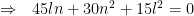$\Rightarrow \,\,\,\, 45ln + 30{n^2} + 15{l^2} = 0$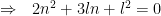$\Rightarrow \,\,\,\, 2{n^2} + 3ln + {l^2} = 0$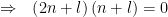$\Rightarrow \,\,\,\, \left( {2n + l} \right)\left( {n + l} \right) = 0$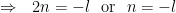$\Rightarrow \,\,\,\, 2n = - l\,\,\,\,{\rm{or}}\,\,\,\,n = - l$
For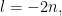$l = - 2n,$ we obtain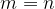$m = n$. A set of direction ratios of one line is therefore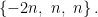$\left\{ { - 2n,\,\,n,\,\,n} \right\}.$
For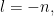$l = - n,$ we obtain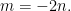$m = - 2n.$ A set of direction ratios of the other line is therefore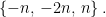$\left\{ { - n,\, - 2n,\,n} \right\}.$
Using the result of example$6$ (the one that you were asked to prove at the end of the question), the angle between the two lines can now be evaluated to be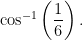${\cos ^{ - 1}}\left( {\dfrac{1}{6}} \right).$